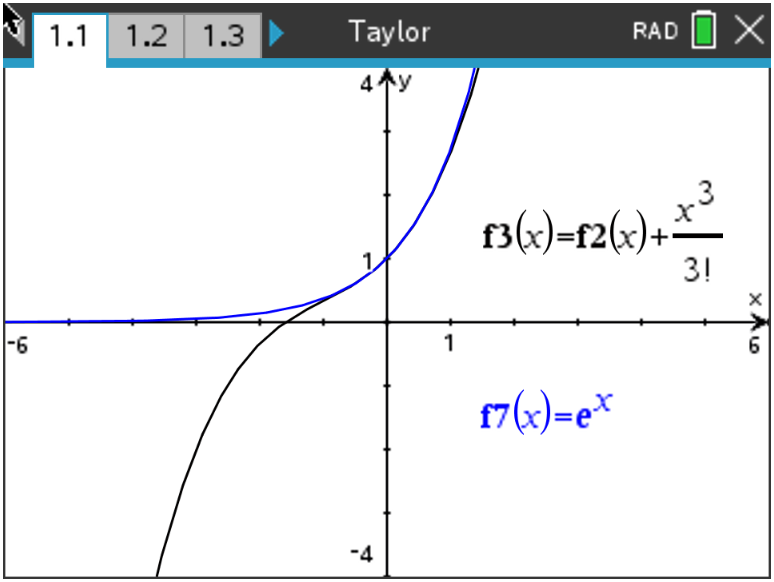Activities

•• Subject Area

• Math: Calculus: Series and Taylor Polynomials

9-12

50 Minutes

• Device
•TI-Nspire™ CX/CX II
•TI-Nspire™ CX CAS/CX II CAS
• Software

TI-Nspire™ CX
TI-Nspire™ CX CAS

5.0

Taylor Polynomials ExplorationActivity Overview

In this activity, students will examine another kind of polynomial approximation that is a generalization of the tangent line approximation. These polynomials are called Taylor polynomials.

Objectives

Students will:

• Define a Taylor polynomial approximation to a function f of degree n about a point x = a.
• Graph convergence of Taylor polynomials.
• Use Taylor polynomials to approximate function values.

Vocabulary

• Taylor polynomial
• factorial
• derivatives